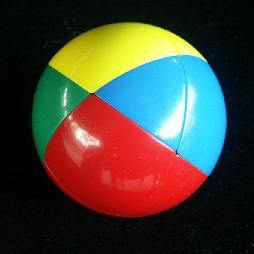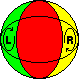# Beachball SkewbThe Beachball Skewb is a sphere which is bisected 4 ways, by planes through the centre of the ball, which splits the surface of the ball up into 8 triangles and 6 squares. Each half of the puzzle has a triangle at its centre and can rotate 120 degrees in either direction. When solved, the surface of the ball is divided into four segments, one colour each, along two of the cutting planes.

The beachball uses the same mechanism as the Skewb, and can therefore be solved in much the same way, but as this puzzle is so much simpler it is better to have a faster solution that is specifically geared to it.

Note that turning one triangle has the same effect on the puzzle as turning the opposite triangle the other way. Therefore the puzzle can be solved by only using one of each pair of opposite triangles. Internally 4 of the triangles are fixed on axes, and the other 4 are moving pieces. Externally there is no visible difference between the two sets.

The puzzle ball was patented by Uwe Meffert, 25 October 1994, US 5,358,247.

## The number of positions:

There are 4 fixed triangles, 4 free triangles, and 6 squares giving a maximum of 6! 4! positions. This limit is not reached because
• There are two pairs of identical squares (4)
• The free triangles must have an even permutation (2)

This leaves 6!·3 = 2,160 positions.

A computer search gave the following result:
MovesPositions
01
18
248
3252
4930
5884
637
Total2,160

This shows that any position can be solved in at most 6 moves (4.2694 on average). In Sloane's On-Line Encyclopedia of Integer Sequences this is included as sequence A079763.

## Solution:

Phase 1: Solve a small segment.

1. First find out the colour of one of the small segments. Simply look at the squares, and find a colour of which there is only one square.
2. If the square is not already adjacent to a triangle of the same colour, then do any move to make it so.
3. Turn the half containing the square and its adjacent triangle so that the square lies between the two triangles of the same colour, thus completing the small segment.

Phase 2: Solve the second small segment

1. Hold the puzzle so that the solved segment is vertical at the back. From now the only moves will be turns of the front left and front right halves because they do not move this solved segment. This restriction does mean the solution uses more moves, but it is much simpler.
2. Find out the colour of the second small segment, i.e. the colour of which there is only one square left. This segment will be solved directly at the front, in the intersection of the two halves that can still turn.
3. If the square is not already adjacent to a triangle of the same colour, then do any move to make it so.
4. Turn the half containing the square and its adjacent triangle so that they do not lie in the intersection of the two ball halves.
5. Turn the other half to join up the other triangle, and so form the second segment.
6. Turn half containing the segment to bring it to the front.Notation:
The final phase is easier to describe with a little bit of notation. Let L denote a clockwise 120 degree turn of the front left half of the ball, and let R be such a turn of the front right half. Anti-clockwise turns of those halves are denoted by L' and R'.

Phase 3: Solve the two large segments.

1. Examine the two triangles in the left unsolved segment. If they are not the same colour, then do RLRLR'LR'. Note how this sequence swaps the two triangles of the front segment. That automatically causes two other triangles to be swapped as required.
2. If all four remaining squares are incorrect, then do the sequence L'R'LRLRLRLR'L'.
3. If two of the squares need to be swapped, then turn the whole ball so that the two small segments are still vertical at the front and back, and the left bottom square correctly matches the left triangles. There are two possibilities for the square belonging at the top left:
1. If the square lies at the top right, do L'R'LRLR'.
2. If the square lies at the bottom right, do RL'R'L'RL.
Note that these are inverses of each other.